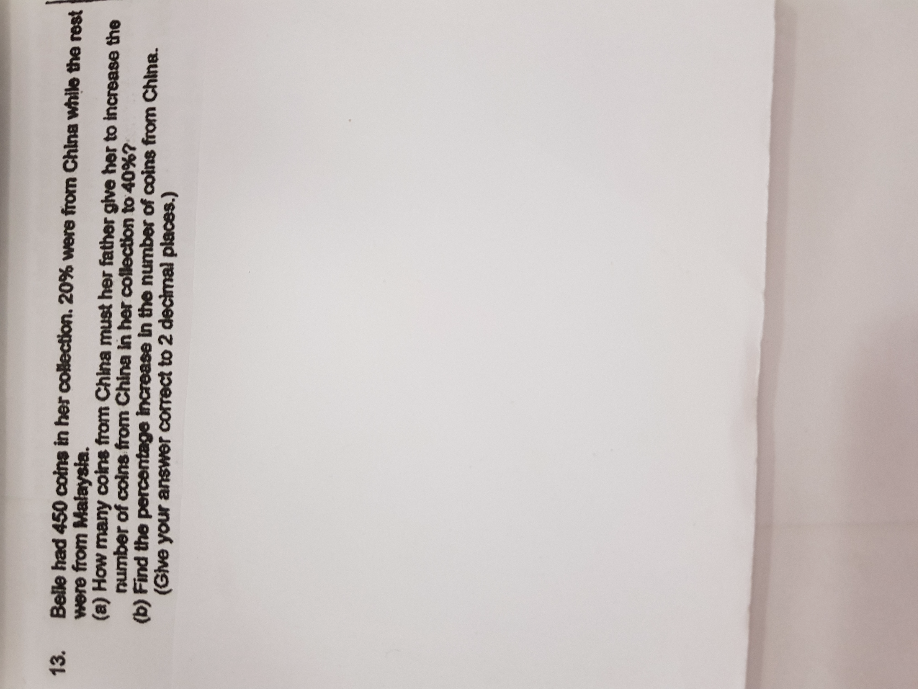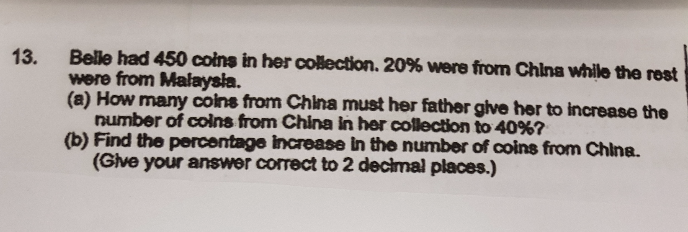# QuestionHi, can anyone help with this qns pls. ThanksTotal -> 450

China (20%) -> 20% × 450 = 90

Malaysia (80%) -> 450 – 90 = 360

China coins increased to 40% means Malaysia dropped to 60% without changing quantity.

60% -> 360

10% -> 360 ÷ 6 = 60

40% -> 60 × 4 = 240

(a) Father gave Belle (240 – 90) = 150 China coins

(b) Percentage increase -> 150/90 × 100 = 166.67% (correct to 2 decimal places)

0 Replies 1 Like